# Count Attendance and Absence with COUNTIF function

In our campus life and work life, we usually record everyone’s attendance. Today we will introduce you the application of Excel COUNTIF function to count the attendance.

Using a week as an example, we use the following example to show you how to use Excel COUNTIF function to count attendance.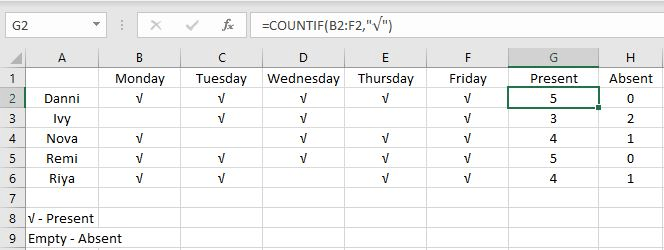As shown above, attendance and absence are counted correctly by Excel COUNTIF function.

## FORMULA

In this example, we have used only the COUNTIF function, without nesting other functions. In a simple case like the one above, we can use COUNTIF function to solve our problem directly.

COUNTIF function belongs to Excel Statistical functions. It counts the number of cells that meet the provided criterion in a certain range.

Syntax:

`=COUNTIF(range, criteria)`

In this example, we can directly apply this function with entering our own range and criteria.

`=COUNTIF(B2:F2,"√") – in G2`

## EXPLANATION

In this example, the “√” in the cell indicates attendance on the corresponding day. Calculating attendance is equivalent to calculating how many ticks there are in B2:F2.

### FULL ATTENDANCE

Use G2 as an example, the range for recording attendance is B2:F2. The condition is “√”. So the formula is =COUNTIF(B2:F2,”√”). We cannot enter “√” in this formula, but we can copy a √ into criteria field and include it in brackets.

In the formula bar, we can expand B2:F2, we can get an array of “√”

`=COUNTIF({"√","√","√","√","√"},"√")`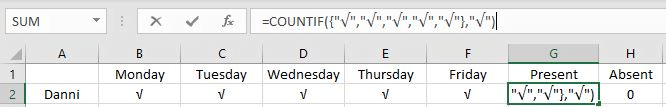Excel COUNTIF function will count the number of times “√” appears in the array.

Obviously, the result is 5.

### PARTIAL ATTANDENCE

Copy down the formula. G3 for example, B3 and E3 cells in the absence of “√”, so in this formula, there are only three “√” in the range.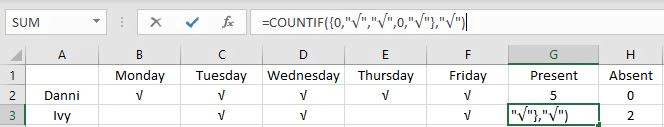After running the formula, the result is 3.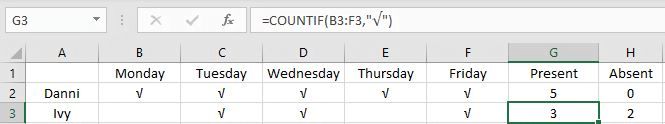### ABSENCE

If the cell is empty, it means it was absent that day. Just replace “√” with “”.

The formula is

`=COUNTIF(B2:F2,"")`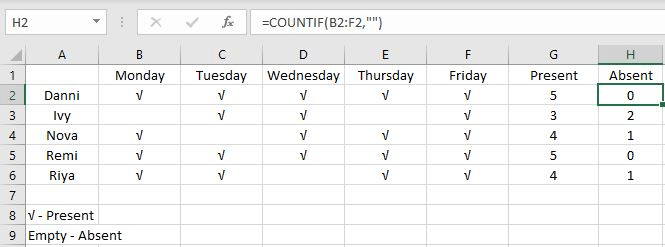Note that there are no spaces between the “”. If we enter a space, such as ” “, we will count how many cells in the range B2:F2 contain spaces.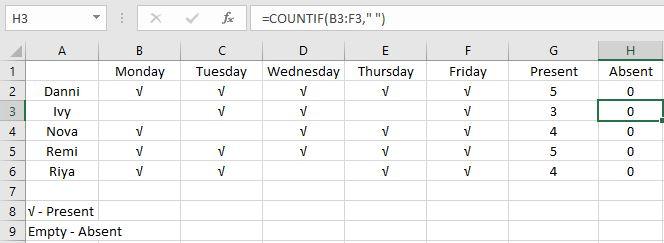### Related Functions

• Excel COUNTIF function
The Excel COUNTIF function will count the number of cells in a range that meet a given criteria. This function can be used to count the different kinds of cells with number, date, text values, blank, non-blanks, or containing specific characters.etc.= COUNTIF (range, criteria)…
Related Posts

Break ties with helper COUNTIF and column

Suppose you got a task to adjust the values that contain the ties; what would be your first attempt to break the ties of the given value? If you are wondering about doing this task manually, let me add that ...

Calculate Total Cost with Excel VLOOKUP Function

In today's article we will show you how to calculate the total cost for a given weight using the Excel VLOOKUP function. This function will help us to find the appropriate unit price for that weight and then we can ...

Excel Formulas To Calculate The Bond Valuation

Assume that you've been assigned a task of calculating bond valuation; so if you are new to Ms Excel or do not have much experience with it, then I am pretty sure about it that doing this task manually might ...

Calculate Cumulative Totals with Excel SUM Function

Today, through a simple example, we will show you how to use one of the most common-used Mathematical functions in excel, the SUM function, to add up the sum. In our daily life, we keep an account of what we ...

BMI Calculation Formula In Ms Excel

You might have come across a task in which you were assigned to make BMI calculations of the supplied numbers, and you may be looking for an efficient approach to accomplish this process rather than doing BMI calculations manually, by ...

Sidebar